# RD Sharma Solutions for Class 9 Maths Chapter 23 Graphical Representation of Statistical Data

## RD Sharma Solutions Class 9 Maths Chapter 23 – Free PDF Download

RD Sharma Solutions for Class 9 Maths Chapter 23 Graphical Representation of Statistical Data is given here which consists of questions and answers related to statistics. In this chapter, students will learn a graphical representation of data. The main objective of providing exercise-wise solutions in PDF is to help students speed up their exam preparation. The solutions are designed based on the latest CBSE Syllabus and exam pattern. Understanding the concepts before solving the exercise-wise problems is very important to obtain a better score in the exam. The students can download solutions that are available in PDF format easily

Graphical representation is a better way to analyze any numerical data. A graph is a type of chart in which the statistical data are represented (In the form of lines or curves). Graphs help us to understand the relationship between variables and measure the position or values of one variable when the other variable is changed by a certain value. The RD Sharma Solutions Class 9 given here explains the solutions in a descriptive manner from Chapter 23. Click on the link below to access all the solutions.

## Download PDF of RD Sharma Solutions for Class 9 Maths Chapter 23 Graphical Representation of Statistical Data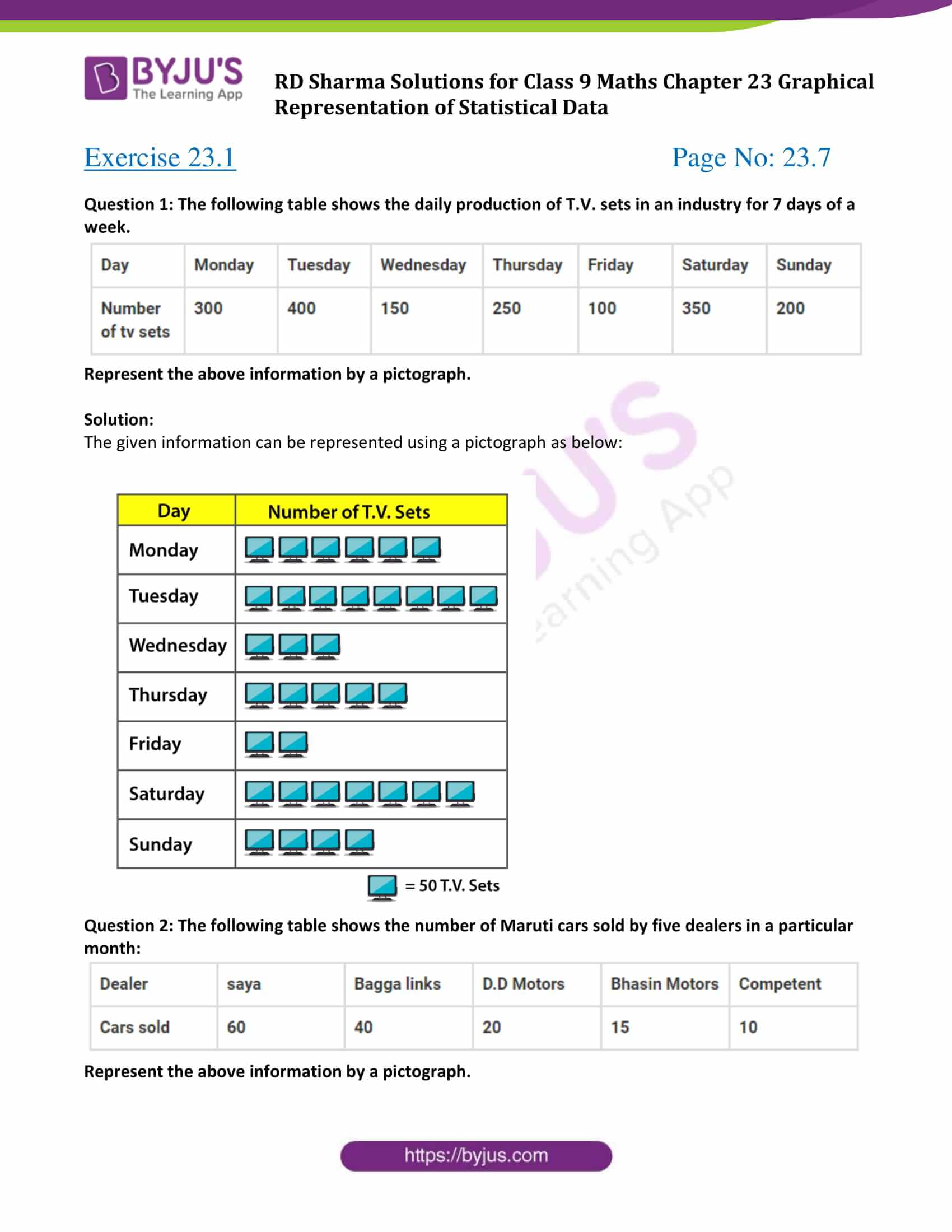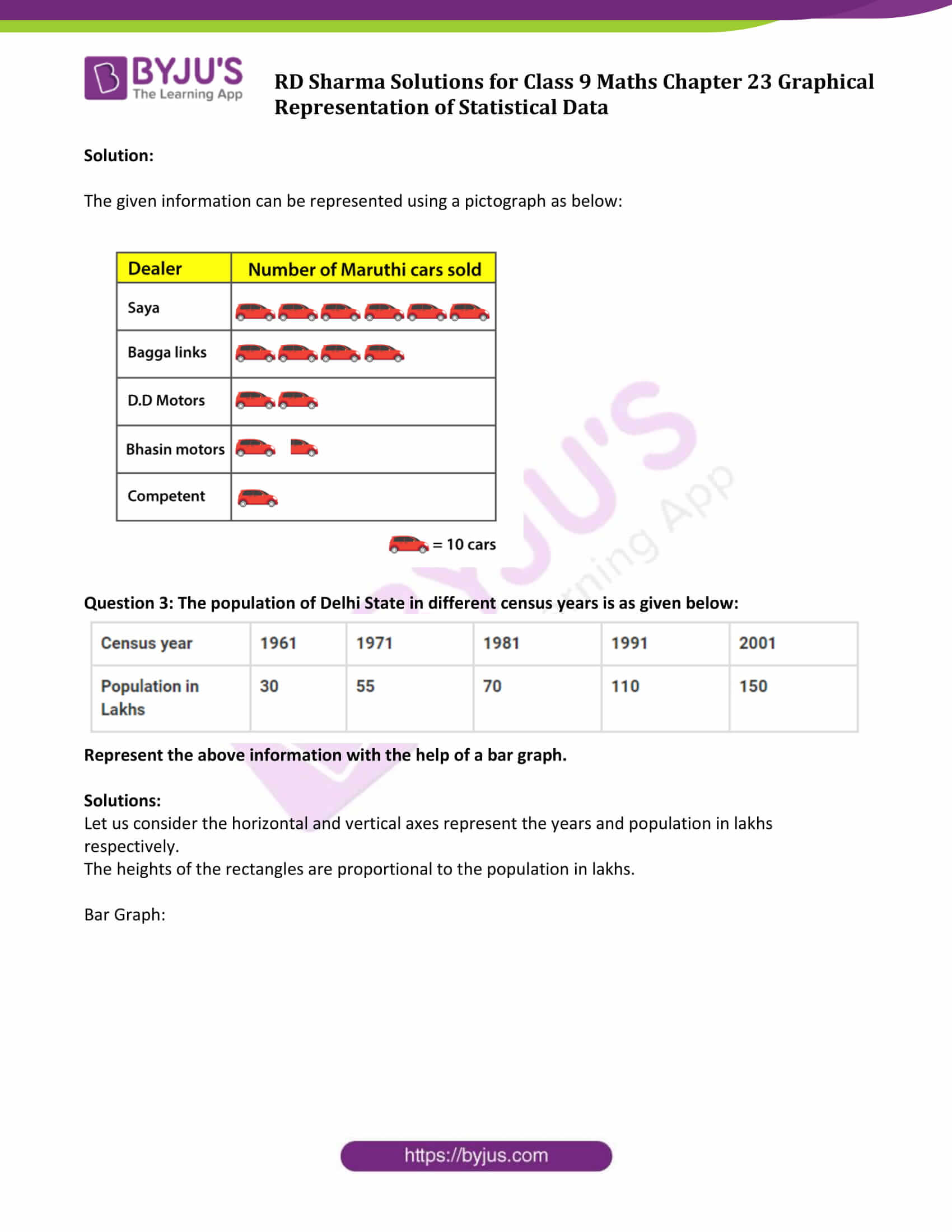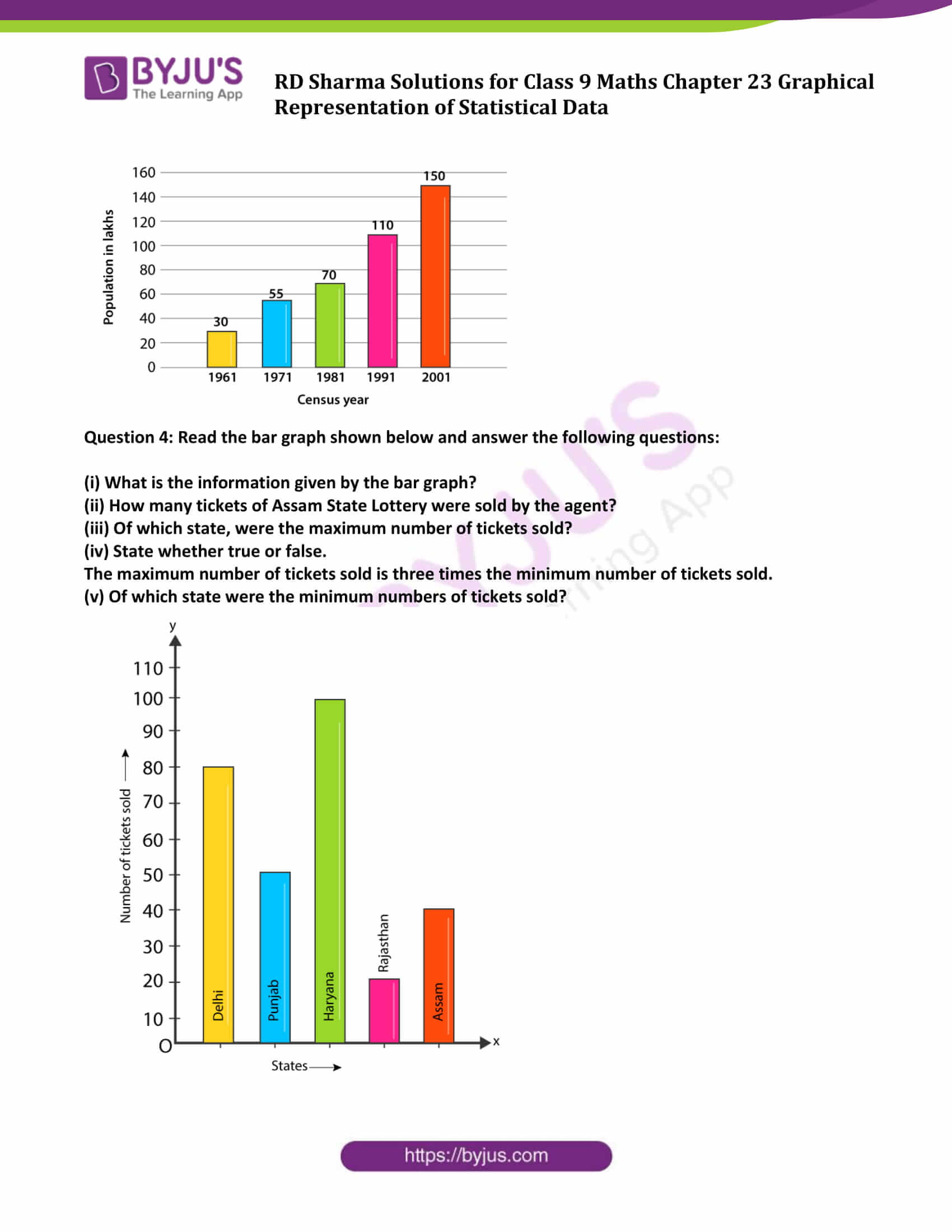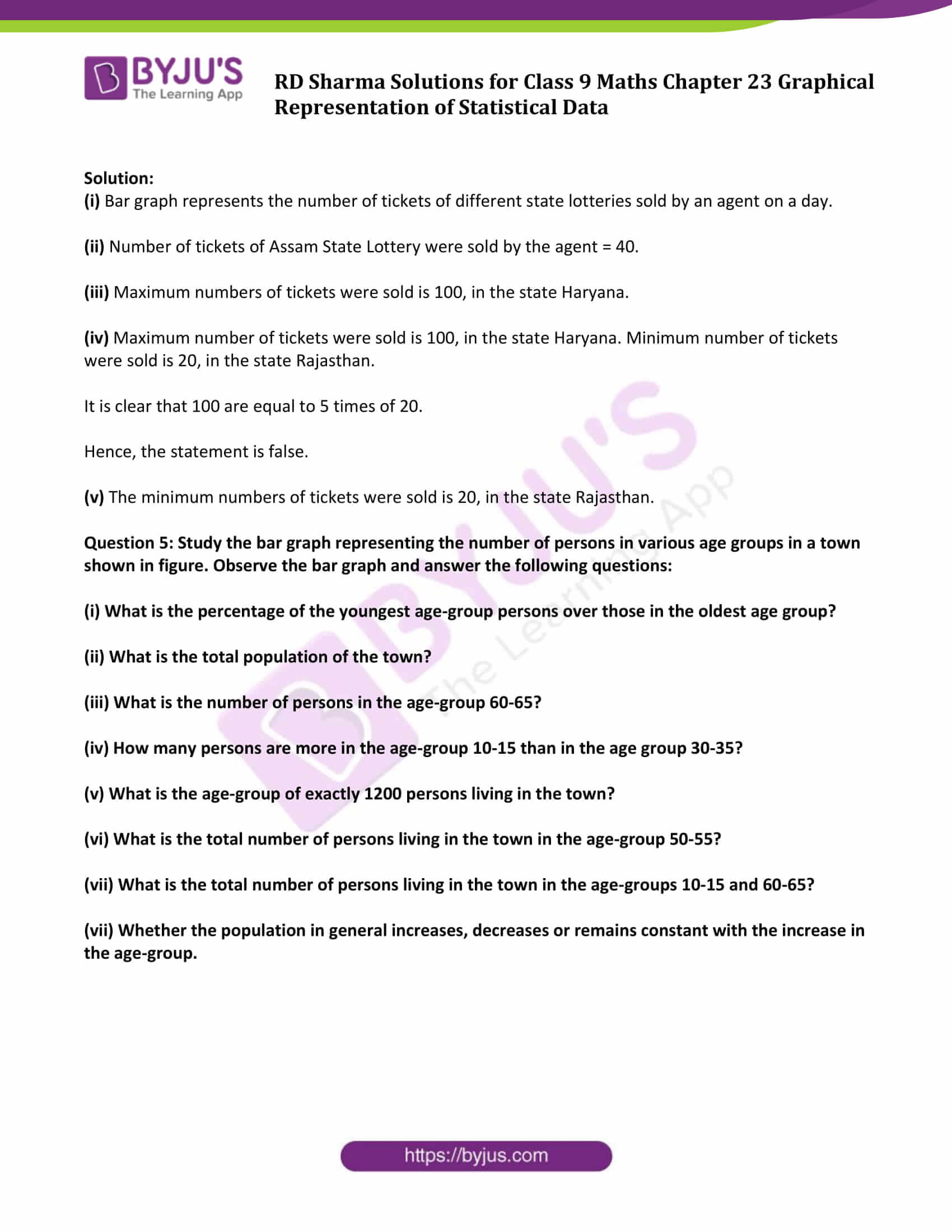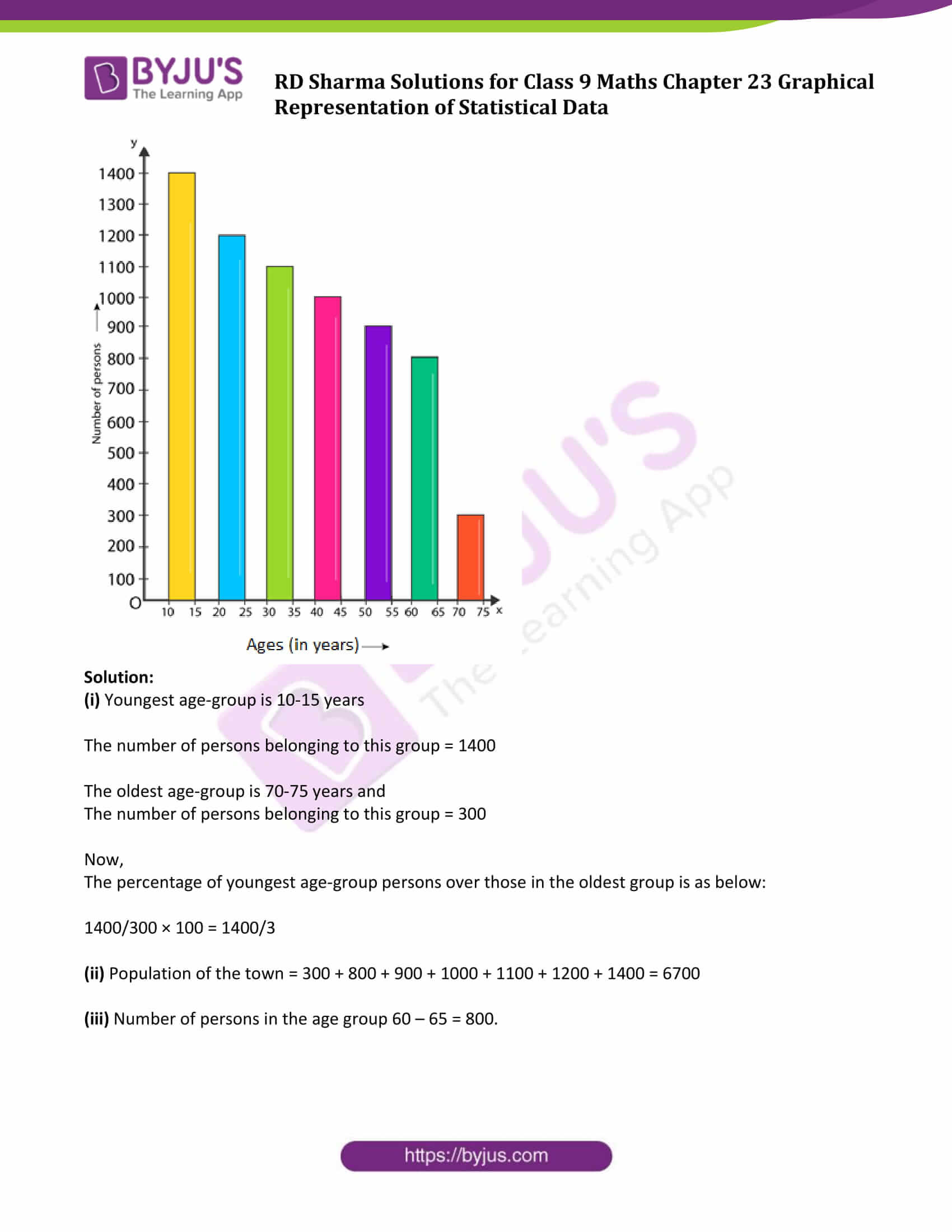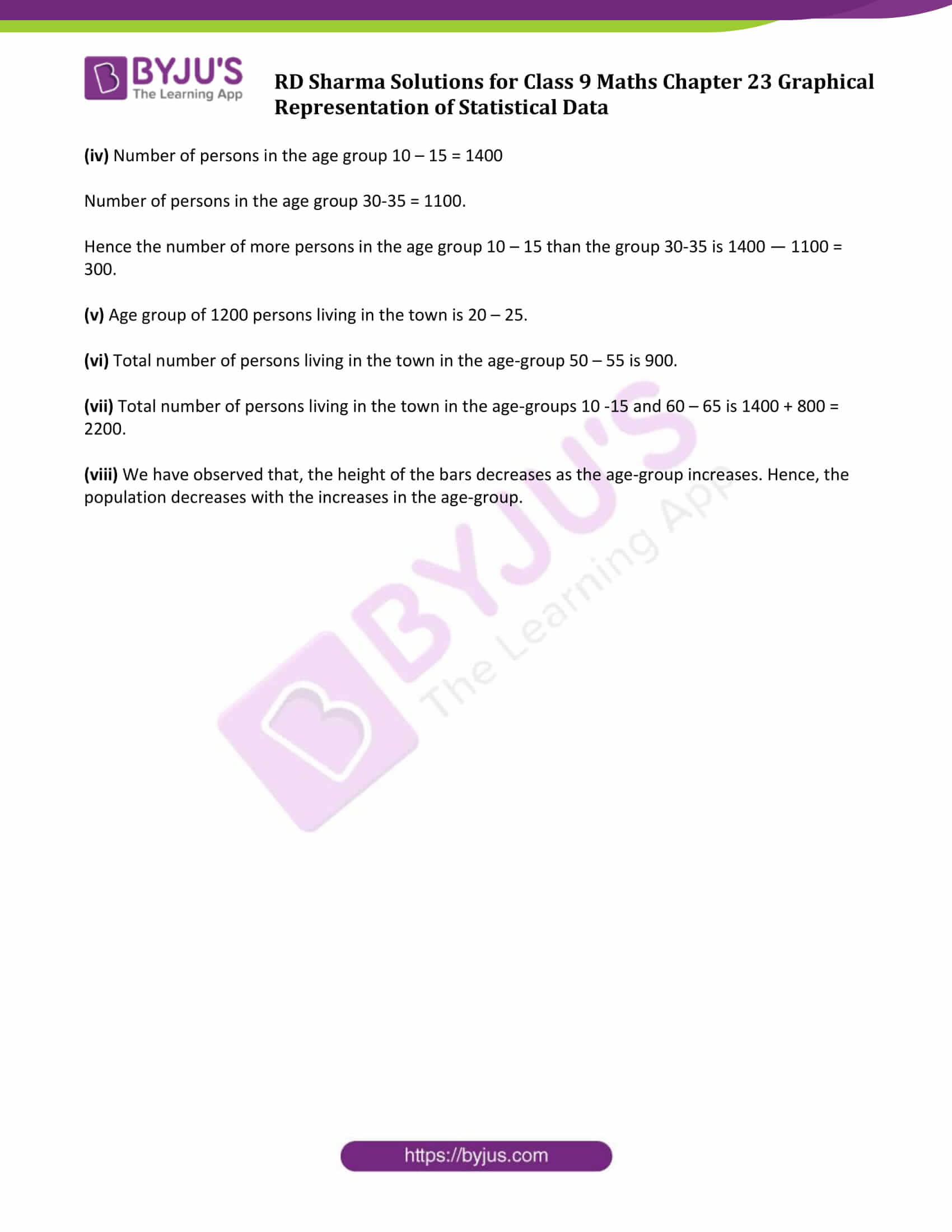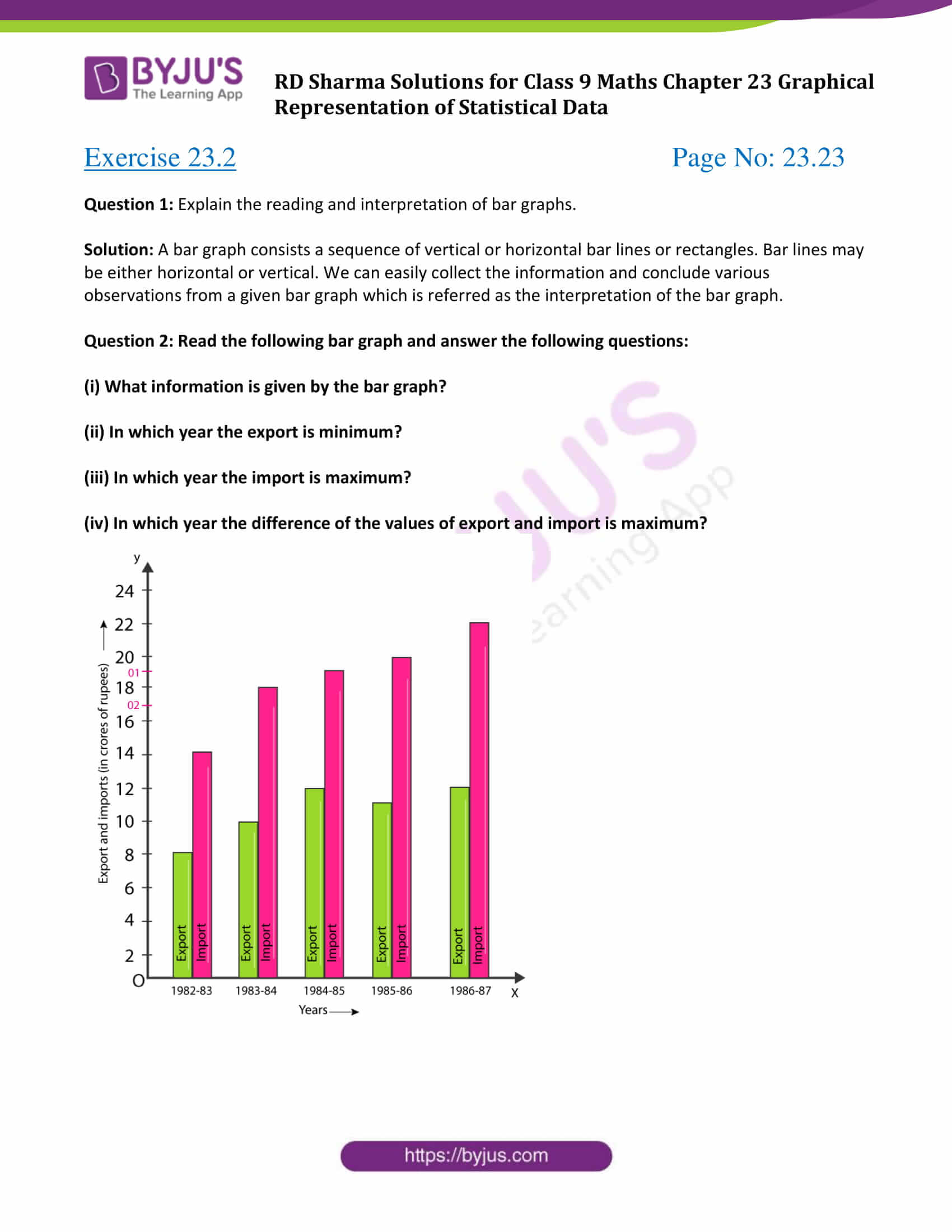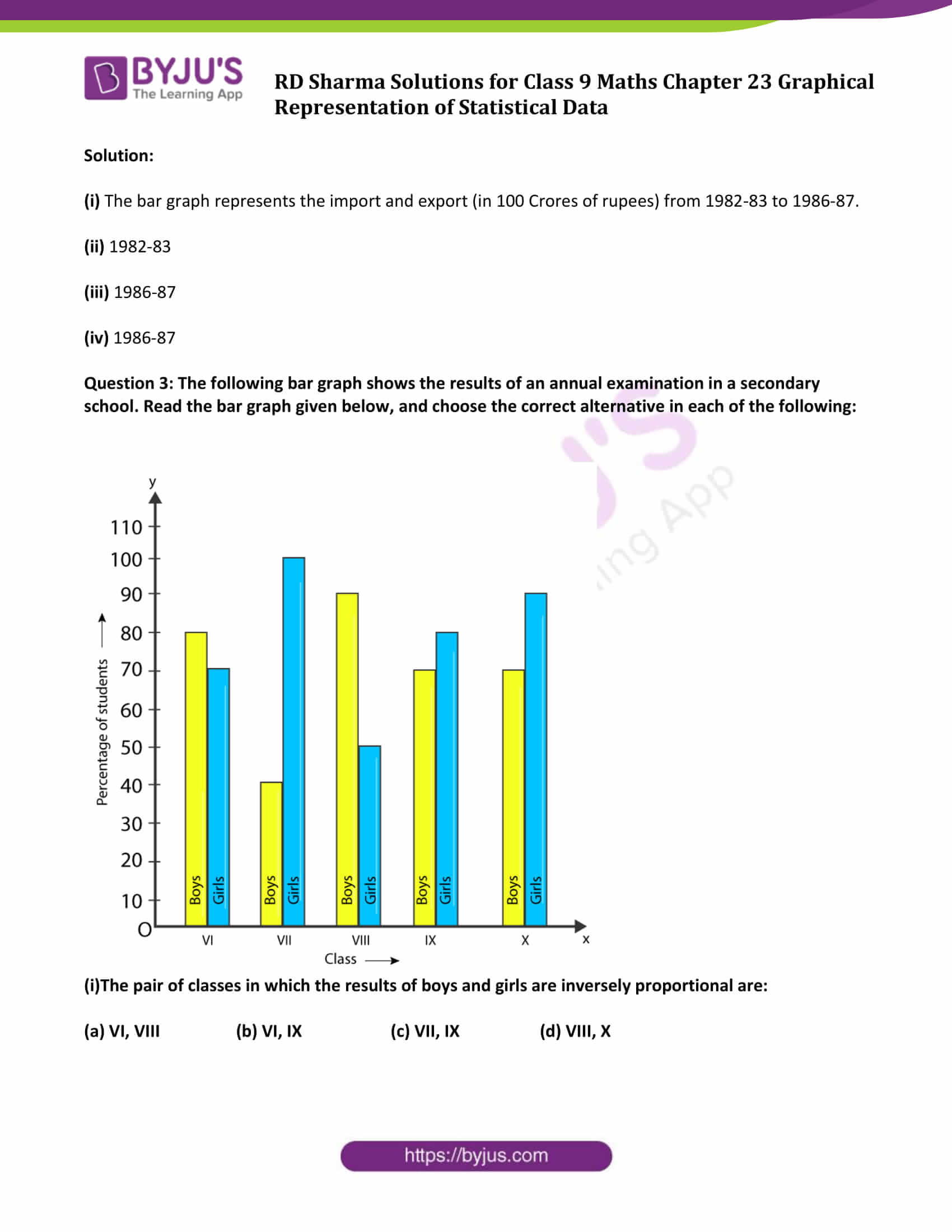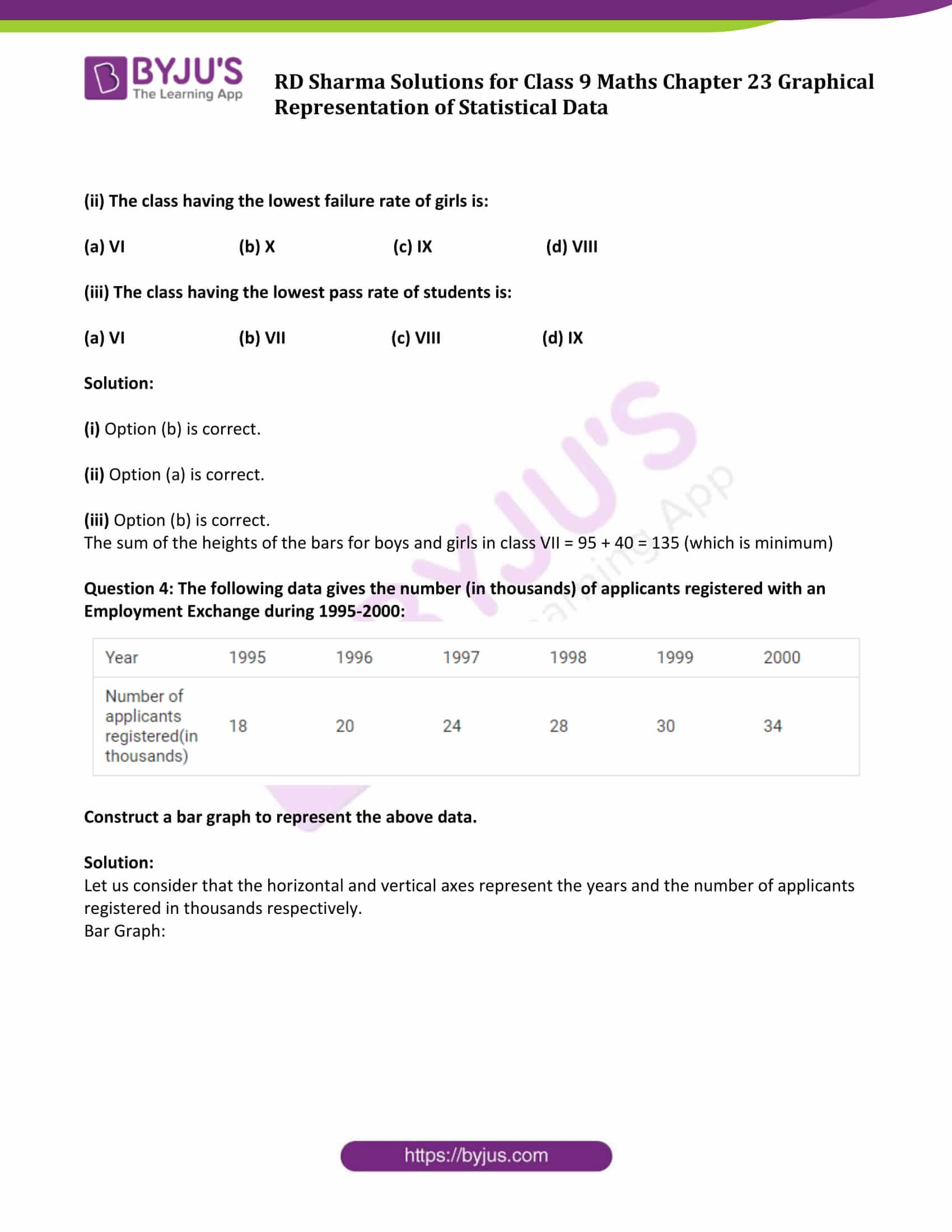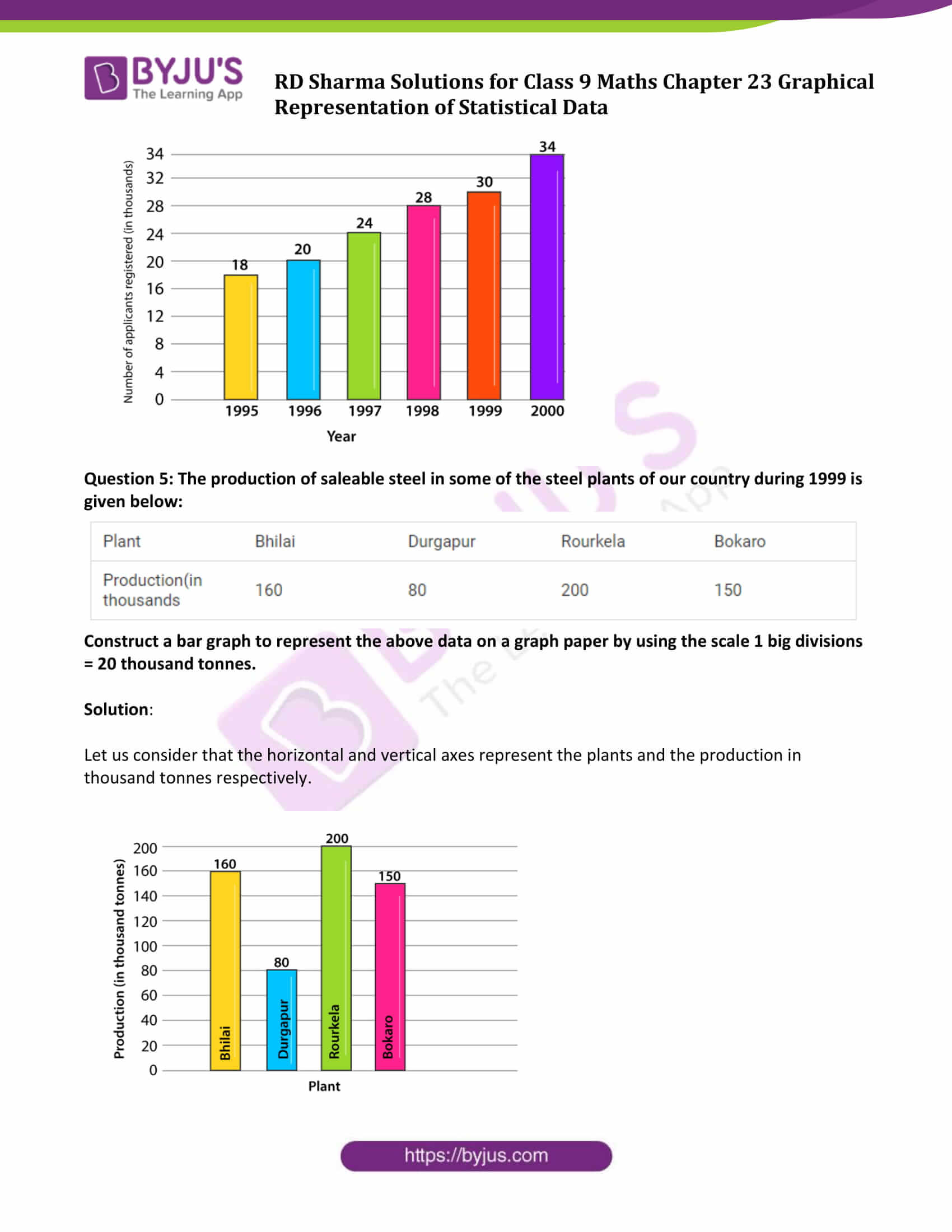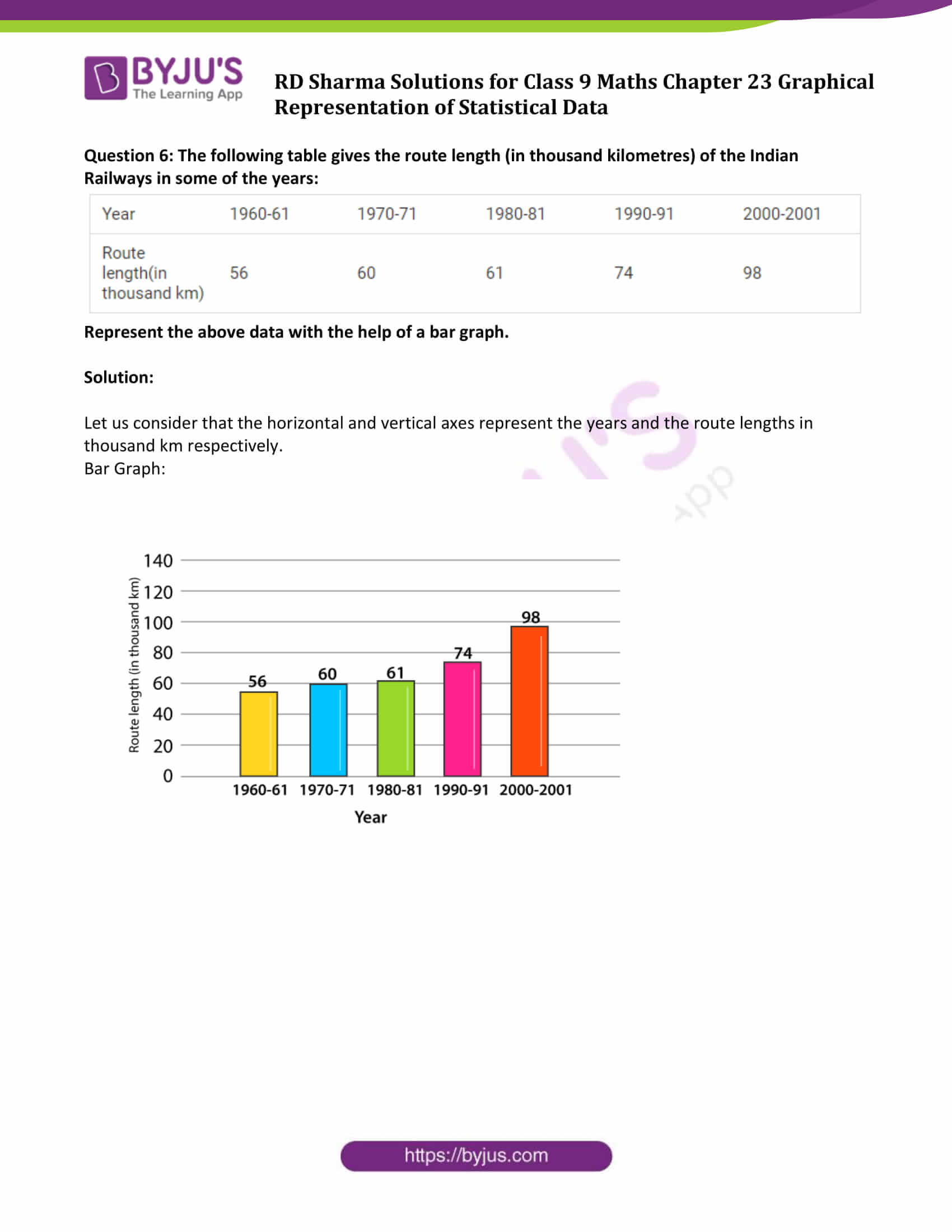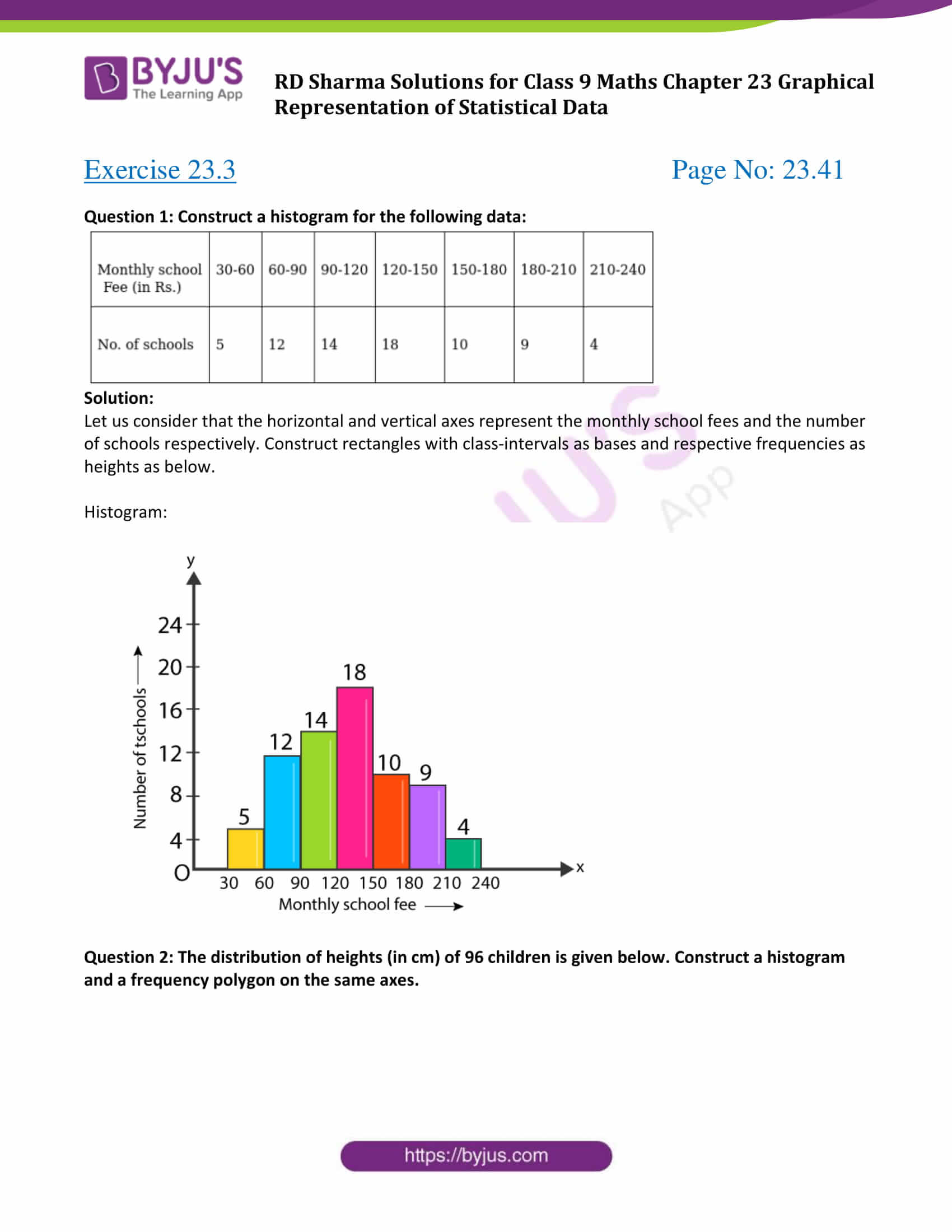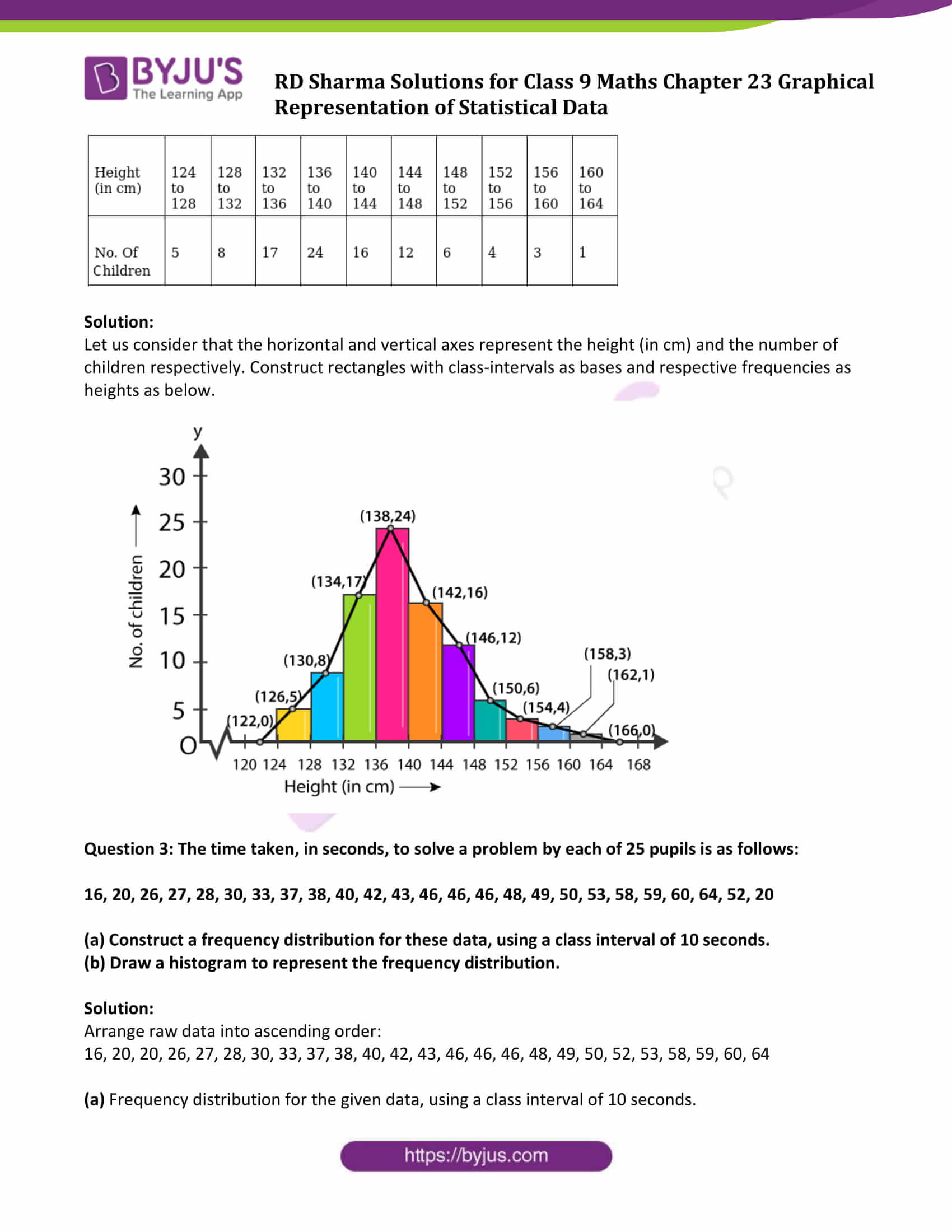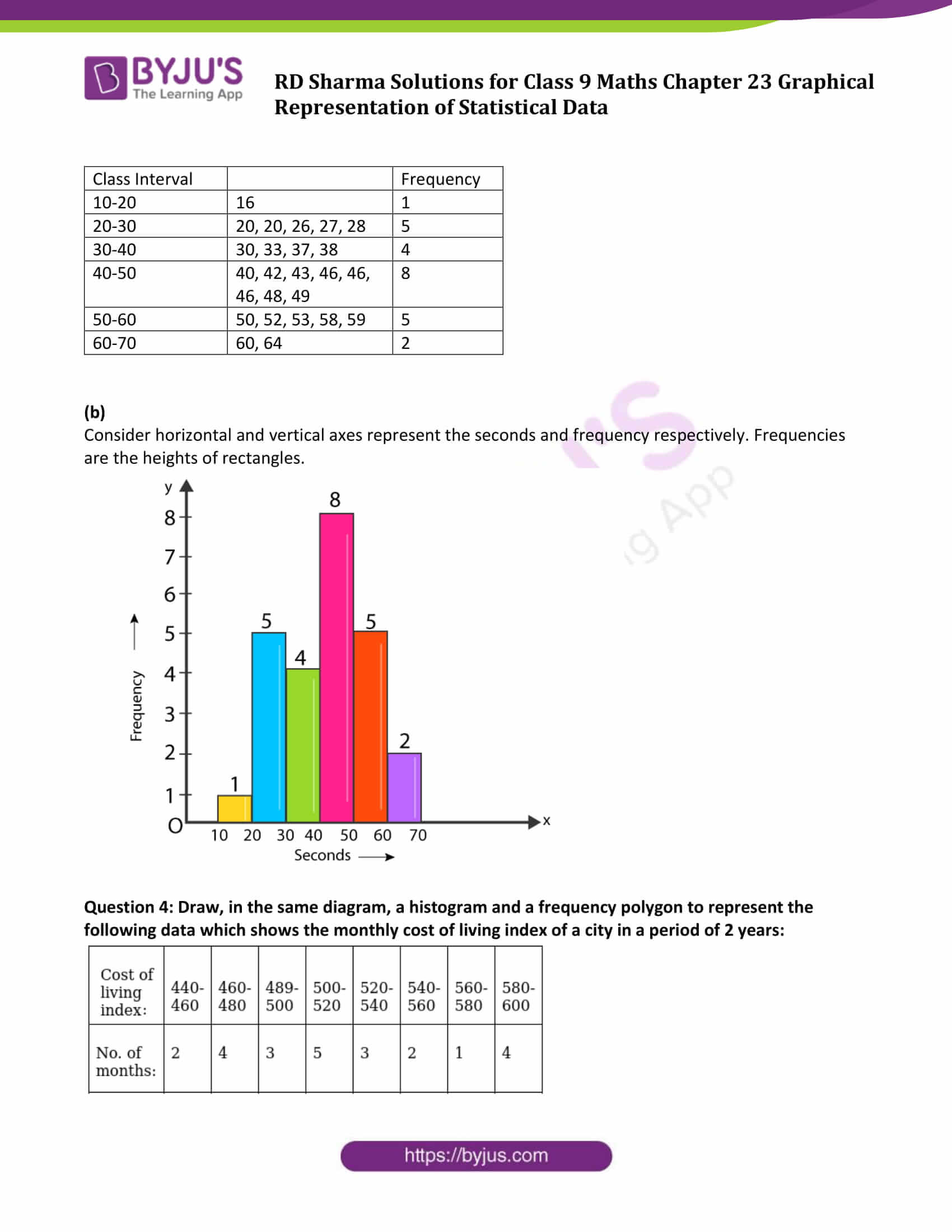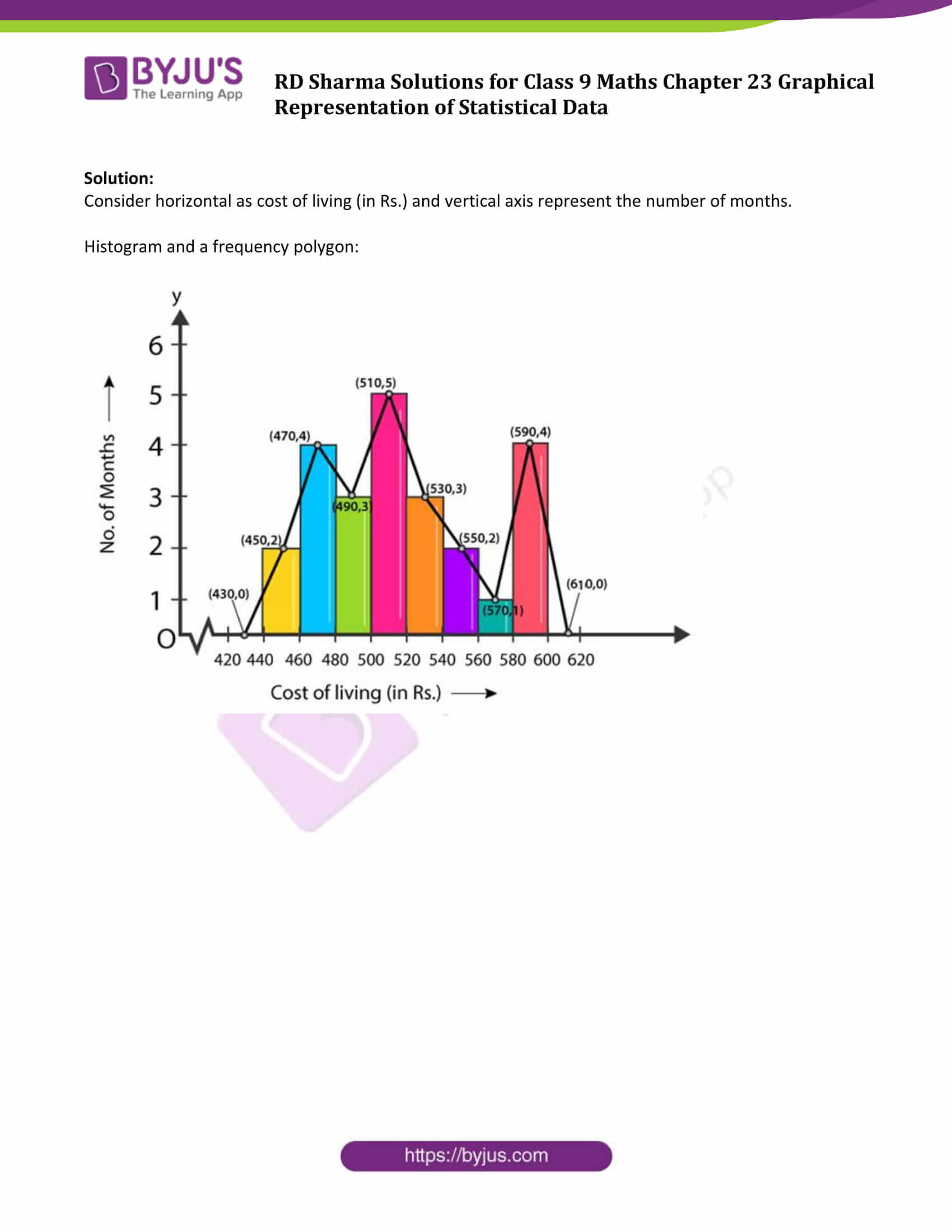### Exercise 23.1 Page No: 23.7

Question 1: The following table shows the daily production of T.V. sets in an industry for 7 days of a week.

 Day Monday Tuesday Wednesday Thursday Friday Saturday Sunday Number of tv sets 300 400 150 250 100 350 200

Represent the above information by a pictograph.

Solution:

The given information can be represented using a pictograph as below: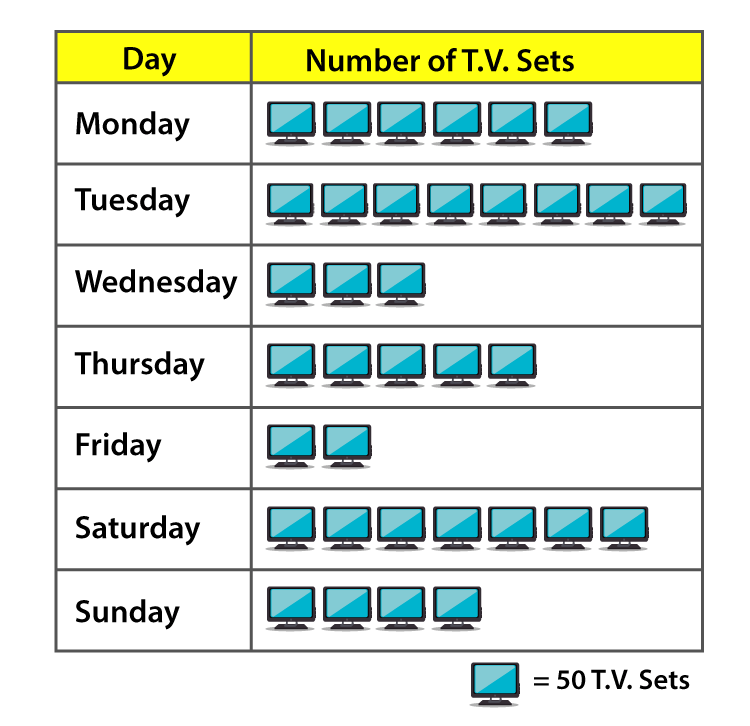Question 2: The following table shows the number of Maruti cars sold by five dealers in a particular month:

 Dealer saya Bagaa links DD motors Bhasin Motors competent cars sold 60 40 20 15 10

Represent the above information by a pictograph.

Solution:

The given information can be represented using a pictograph as below: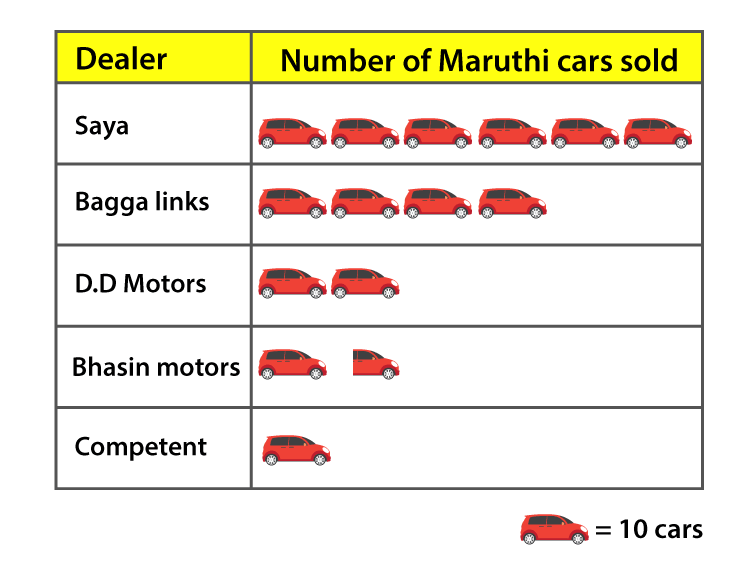Question 3: The population of Delhi State in different census years is as given below:

 Cenus year 1961 1971 1981 1991 2001 Population in lakhs 30 55 70 110 150

Represent the above information with the help of a bar graph.

Solutions:

Let us consider the horizontal and vertical axes represent the years and population in lakhs respectively.

The heights of the rectangles are proportional to the population in lakhs.

Bar Graph: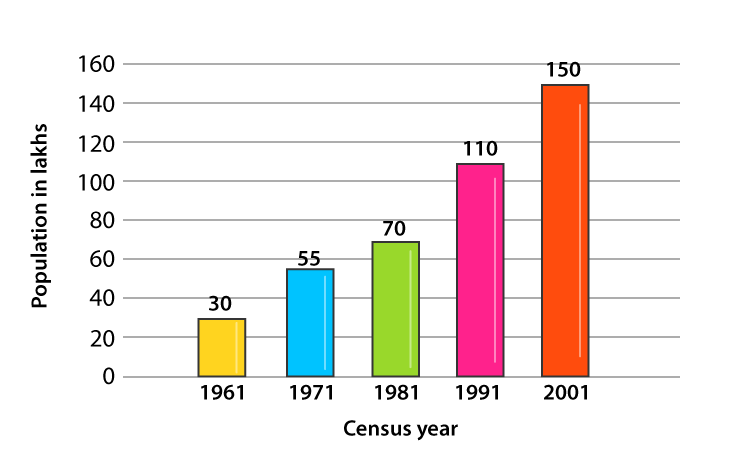Question 4: Read the bar graph shown below and answer the following questions:

(i) What is the information given by the bar graph?

(ii) How many tickets of Assam State Lottery were sold by the agent?

(iii) Of which state, were the maximum number of tickets sold?

(iv) State whether true or false.

The maximum number of tickets sold is three times the minimum number of tickets sold.

(v) Of which state were the minimum numbers of tickets sold?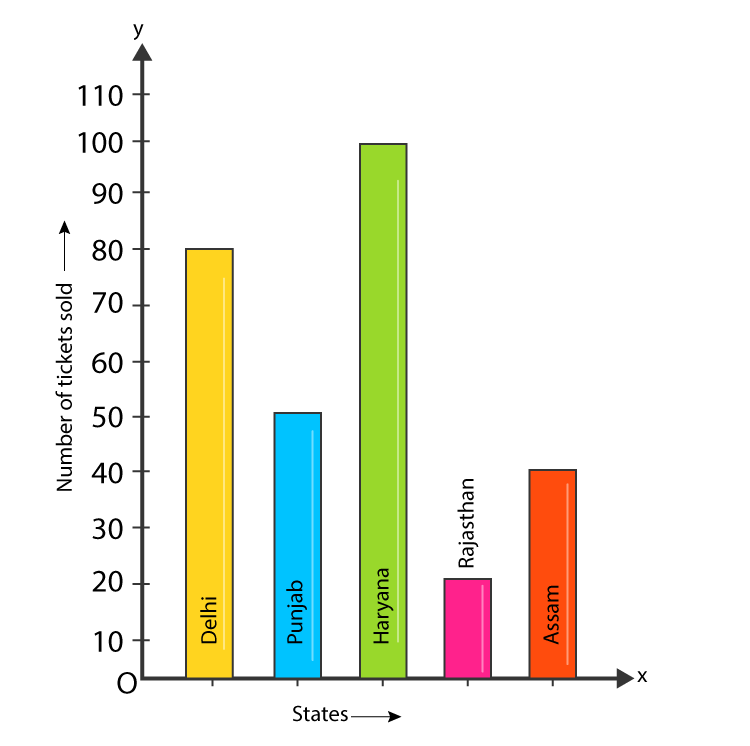Solution:

(i) Bar graph represents the number of tickets of different state lotteries sold by an agent on a day.

(ii) Number of tickets of Assam State Lottery were sold by the agent = 40.

(iii) Maximum numbers of tickets were sold is 100, in the state Haryana.

(iv) Maximum number of tickets were sold is 100, in the state Haryana. Minimum number of tickets were sold is 20, in the state Rajasthan.

It is clear that 100 are equal to 5 times of 20.

Hence, the statement is false.

(v) The minimum numbers of tickets were sold is 20, in the state Rajasthan.

Question 5: Study the bar graph representing the number of persons in various age groups in a town shown in figure. Observe the bar graph and answer the following questions:

(i) What is the percentage of the youngest age-group persons over those in the oldest age group?

(ii) What is the total population of the town?

(iii) What is the number of persons in the age-group 60-65?

(iv) How many persons are more in the age-group 10-15 than in the age group 30-35?

(v) What is the age-group of exactly 1200 persons living in the town?

(vi) What is the total number of persons living in the town in the age-group 50-55?

(vii) What is the total number of persons living in the town in the age-groups 10-15 and 60-65?

(vii) Whether the population in general increases, decreases or remains constant with the increase in the age-group.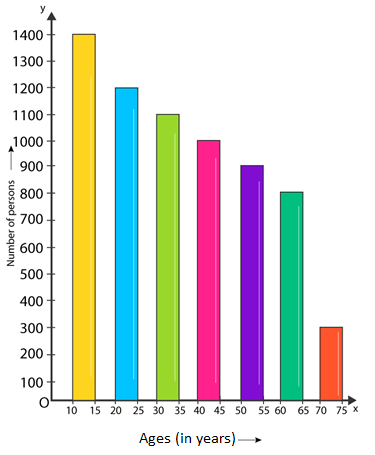Solution:

(i) Youngest age-group is 10-15 years

The number of persons belonging to this group = 1400

The oldest age-group is 70-75 years and

The number of persons belonging to this group = 300

Now,

The percentage of youngest age-group persons over those in the oldest group is as below:

1400/300 × 100 = 1400/3

(ii) Population of the town = 300 + 800 + 900 + 1000 + 1100 + 1200 + 1400 = 6700

(iii) Number of persons in the age group 60 – 65 = 800.

(iv) Number of persons in the age group 10 – 15 = 1400

Number of persons in the age group 30-35 = 1100.

Hence the number of more persons in the age group 10 – 15 than the group 30-35 is 1400 — 1100 = 300.

(v) Age group of 1200 persons living in the town is 20 – 25.

(vi) Total number of persons living in the town in the age-group 50 – 55 is 900.

(vii) Total number of persons living in the town in the age-groups 10 -15 and 60 – 65 is 1400 + 800 = 2200.

(viii) We have observed that, the height of the bars decreases as the age-group increases. Hence, the population decreases with the increases in the age-group.

### Exercise 23.2 Page No: 23.23

Question 1: Explain the reading and interpretation of bar graphs.

Solution: A bar graph consists a sequence of vertical or horizontal bar lines or rectangles. Bar lines may be either horizontal or vertical. We can easily collect the information and conclude various observations from a given bar graph which is referred as the interpretation of the bar graph.

Question 2: Read the following bar graph and answer the following questions:

(i) What information is given by the bar graph?

(ii) In which year the export is minimum?

(iii) In which year the import is maximum?

(iv) In which year the difference of the values of export and import is maximum?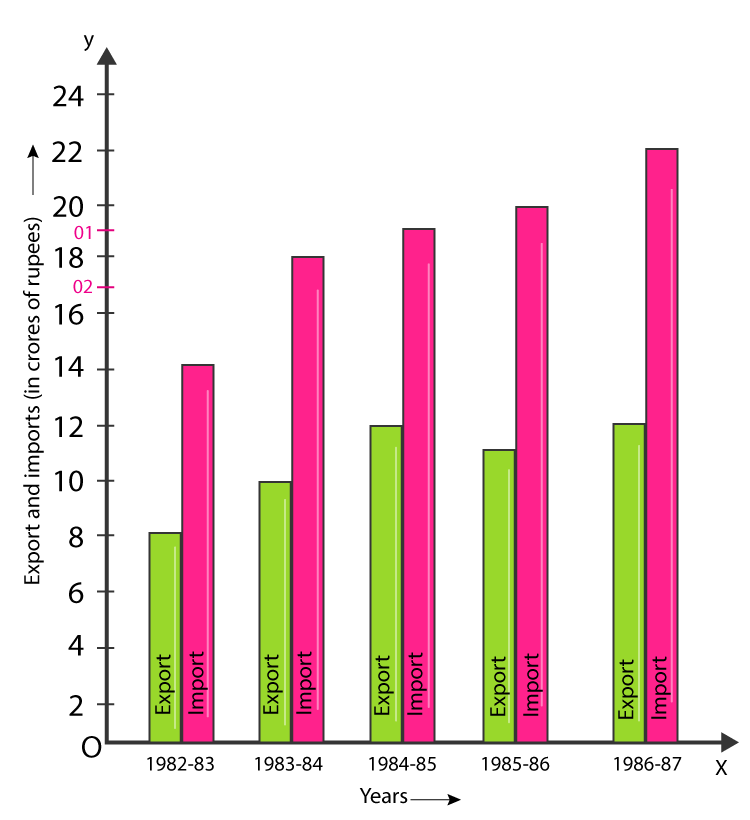Solution:

(i) The bar graph represents the import and export (in 100 Crores of rupees) from 1982-83 to 1986-87.

(ii) 1982-83

(iii) 1986-87

(iv) 1986-87

Question 3: The following bar graph shows the results of an annual examination in a secondary school. Read the bar graph given below, and choose the correct alternative in each of the following: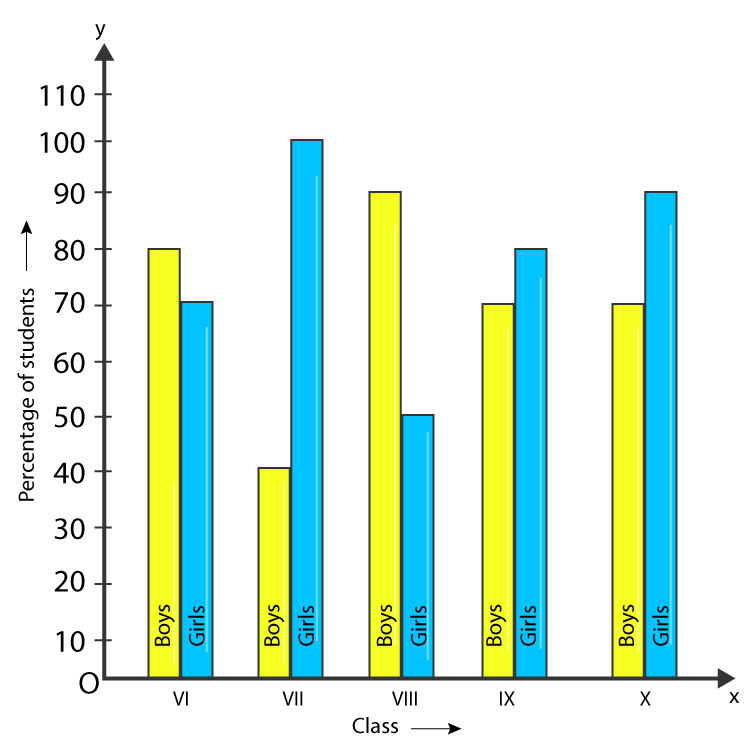(i)The pair of classes in which the results of boys and girls are inversely proportional are:

(a) VI, VIII (b) VI, IX (c) VII, IX (d) VIII, X

(ii) The class having the lowest failure rate of girls is:

(a) VI (b) X (c) IX (d) VIII

(iii) The class having the lowest pass rate of students is:

(a) VI (b) VII (c) VIII (d) IX

Solution:

(i) Option (b) is correct.

(ii) Option (a) is correct.

(iii) Option (b) is correct.

The sum of the heights of the bars for boys and girls in class VII = 95 + 40 = 135 (which is minimum)

Question 4: The following data gives the number (in thousands) of applicants registered with an Employment Exchange during 1995-2000: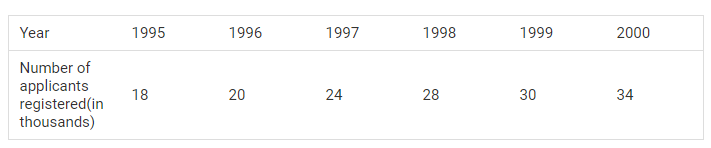Construct a bar graph to represent the above data.

Solution:

Let us consider that the horizontal and vertical axes represent the years and the number of applicants registered in thousands respectively.

Bar Graph: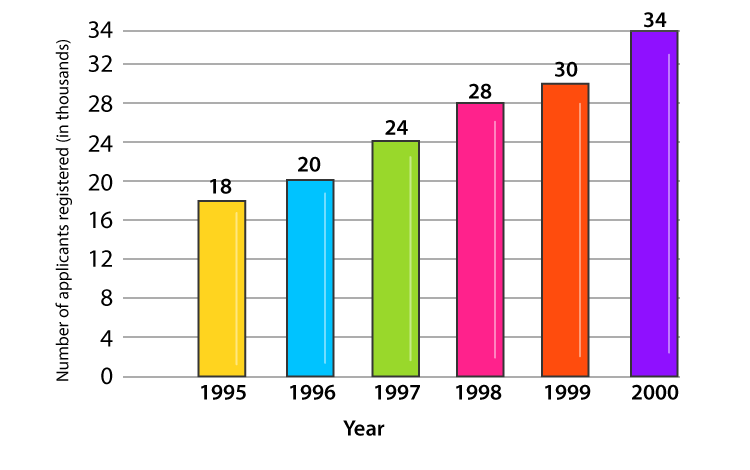Question 5: The production of saleable steel in some of the steel plants of our country during 1999 is given below: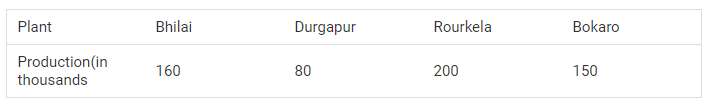Construct a bar graph to represent the above data on a graph paper by using the scale 1 big divisions = 20 thousand tonnes.

Solution:

Let us consider that the horizontal and vertical axes represent the plants and the production in thousand tonnes respectively.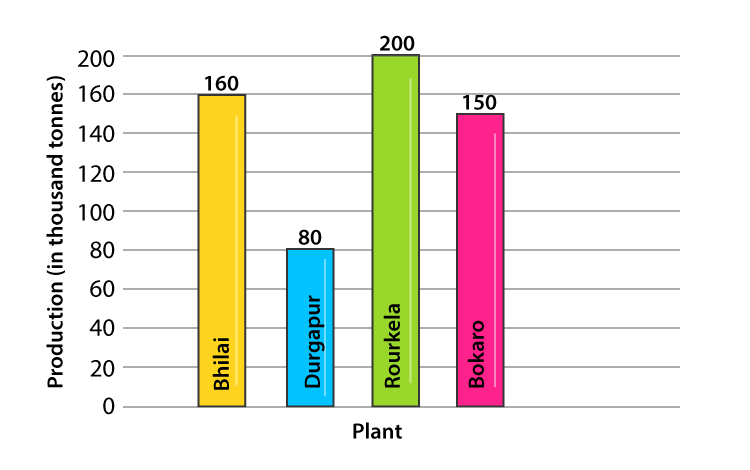Question 6: The following table gives the route length (in thousand kilometres) of the Indian Railways in some of the years:Represent the above data with the help of a bar graph.

Solution:

Let us consider that the horizontal and vertical axes represent the years and the route lengths in thousand km respectively.

Bar Graph: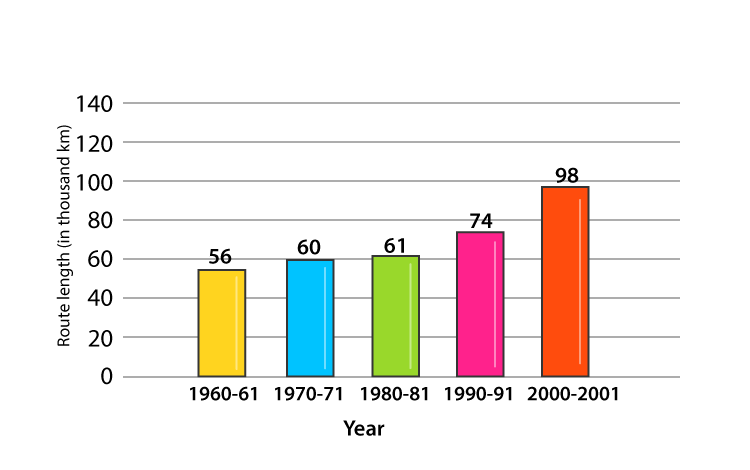### Exercise 23.3 Page No: 23.41

Question 1: Construct a histogram for the following data: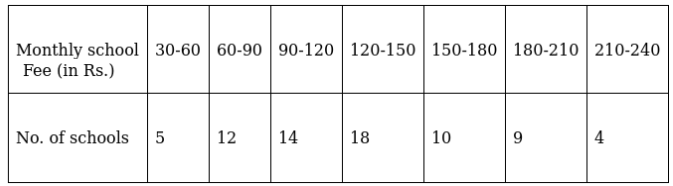Solution:

Let us consider that the horizontal and vertical axes represent the monthly school fees and the number of schools respectively. Construct rectangles with class-intervals as bases and respective frequencies as heights as below.

Histogram: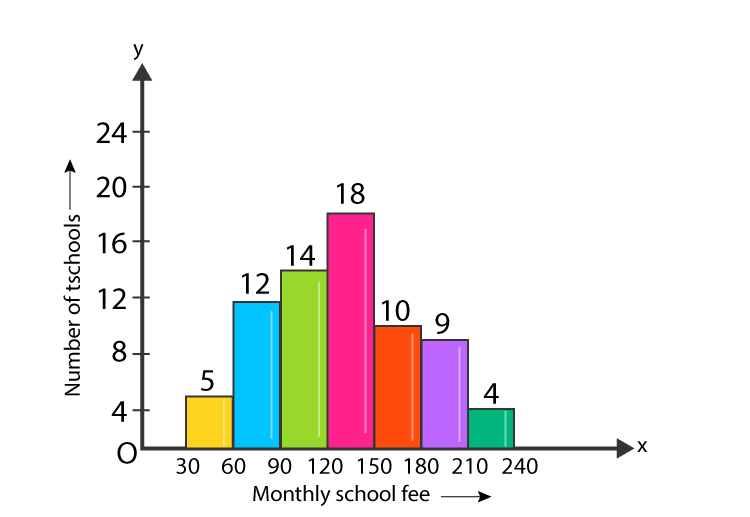Question 2: The distribution of heights (in cm) of 96 children is given below. Construct a histogram and a frequency polygon on the same axes.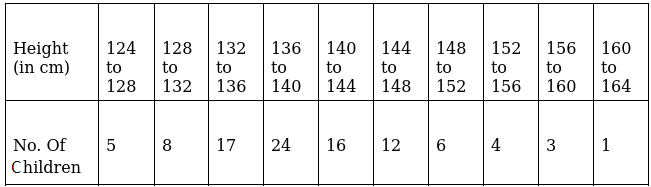Solution:

Let us consider that the horizontal and vertical axes represent the height (in cm) and the number of children respectively. Construct rectangles with class-intervals as bases and respective frequencies as heights as below.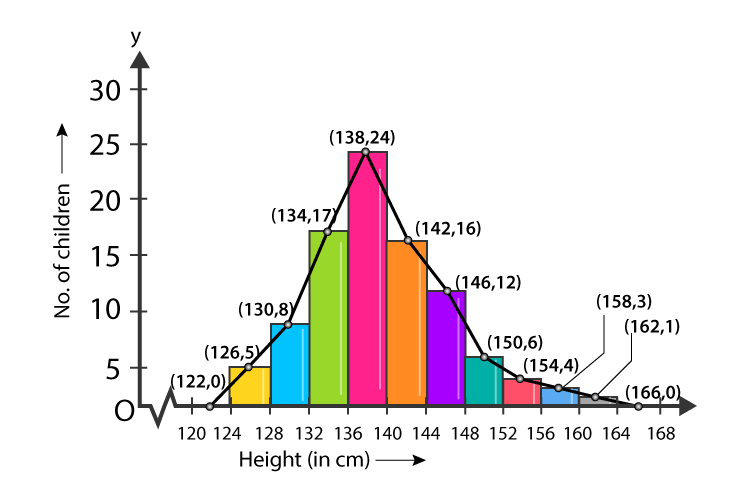Question 3: The time taken, in seconds, to solve a problem by each of 25 pupils is as follows:

16, 20, 26, 27, 28, 30, 33, 37, 38, 40, 42, 43, 46, 46, 46, 48, 49, 50, 53, 58, 59, 60, 64, 52, 20

(a) Construct a frequency distribution for these data, using a class interval of 10 seconds.

(b) Draw a histogram to represent the frequency distribution.

Solution:

Arrange raw data into ascending order:

16, 20, 20, 26, 27, 28, 30, 33, 37, 38, 40, 42, 43, 46, 46, 46, 48, 49, 50, 52, 53, 58, 59, 60, 64

(a) Frequency distribution for the given data, using a class interval of 10 seconds.

 Class Interval Frequency 10-20 16 1 20-30 20, 20, 26, 27, 28 5 30-40 30, 33, 37, 38 4 40-50 40, 42, 43, 46, 46, 46, 48, 49 8 50-60 50, 52, 53, 58, 59 5 60-70 60, 64 2

(b)

Consider horizontal and vertical axes represent the seconds and frequency respectively. Frequencies are the heights of rectangles.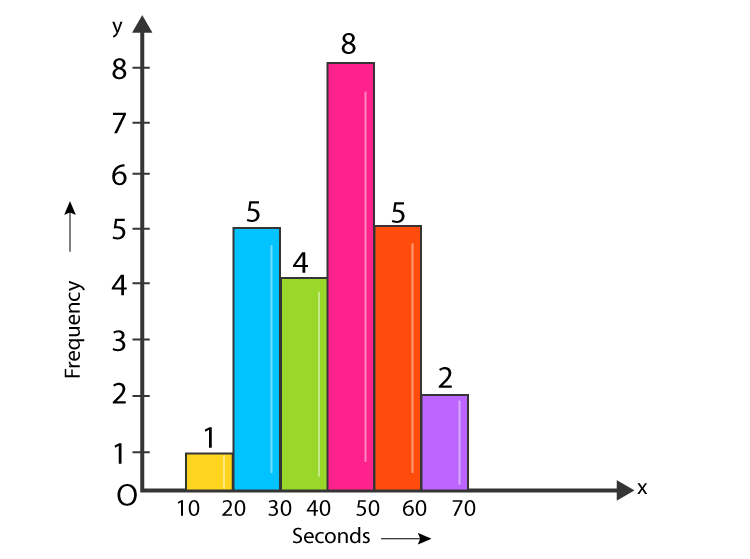Question 4: Draw, in the same diagram, a histogram and a frequency polygon to represent the following data which shows the monthly cost of living index of a city in a period of 2 years: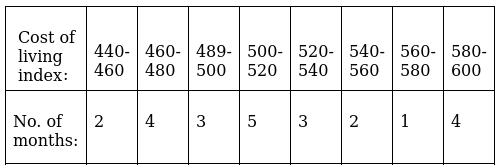Solution:

Consider horizontal as cost of living (in Rs.) and vertical axis represent the number of months.

Histogram and a frequency polygon: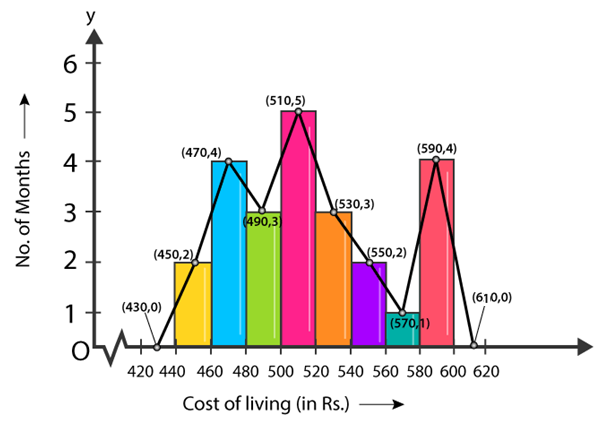### RD Sharma Solutions for Class 9 Maths Chapter 23 Graphical Representation of Statistical Data

In the 23rd Chapter of Class 9 RD Sharma Solutions students will study important concepts listed below:

• Graphical Representation of Statistical Data introduction
• Bar Graph
• Histogram
• Frequency polygon and its algorithm and many more

## Frequently Asked Questions on RD Sharma Solutions for Class 9 Maths Chapter 23

### What are the key benefits of following RD Sharma Solutions for Class 9 Maths Chapter 23?

Students who are in search of the best study material can opt for RD Sharma Solutions as they provide a thorough knowledge of concepts in an efficient manner. Regular practice of these solutions helps students to clear their doubts quickly and boosts confidence to face the exam without any fear. Students can make use of these solutions in PDF format at BYJU’S as per their requirements.

### List out the important concepts discussed in RD Sharma Solutions for Class 9 Maths Chapter 23.

The main concepts covered in RD Sharma Solutions for Class 9 Maths Chapter 23 are listed below:
1. Graphical Representation of Statistical Data introduction
2. Bar Graph
3. Histogram
4. Frequency polygon and its algorithm and many more

### How BYJU’S help students in learning RD Sharma Solutions for Class 9 Maths Chapter 23?

BYJU’S website provides accurate and detailed solutions for all questions provided in the RD Sharma textbook. The solutions are designed by our subject experts to facilitate a smooth and precise understanding of concepts. RD Sharma Solutions offer in-depth knowledge of each concept in a simple and understandable language as per the student’s intelligence quotient. Diligent practice of these solutions boosts confidence in solving complex problems effortlessly among students.